# Resources tagged with: Place value

Filter by: Content type:
Age range:
Challenge level:

There are 125 NRICH Mathematical resources connected to Place value, you may find related items under The Number System and Place Value.

Broad Topics > The Number System and Place Value > Place value##### Age 5 to 11Challenge Level

Try out this number trick. What happens with different starting numbers? What do you notice?##### Age 5 to 11Challenge Level

Who said that adding couldn't be fun?### Subtraction Surprise

##### Age 7 to 14Challenge Level

Try out some calculations. Are you surprised by the results?### Multiply Multiples 3

##### Age 7 to 11Challenge Level

Have a go at balancing this equation. Can you find different ways of doing it?### Round the Dice Decimals 1

##### Age 7 to 11Challenge Level

Use two dice to generate two numbers with one decimal place. What happens when you round these numbers to the nearest whole number?### Round the Dice Decimals 2

##### Age 7 to 11Challenge Level

What happens when you round these numbers to the nearest whole number?### Multiply Multiples 2

##### Age 7 to 11Challenge Level

Can you work out some different ways to balance this equation?### Multiply Multiples 1

##### Age 7 to 11Challenge Level

Can you complete this calculation by filling in the missing numbers? In how many different ways can you do it?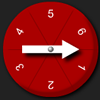### Two Spinners

##### Age 5 to 7Challenge Level

What two-digit numbers can you make with these two dice? What can't you make?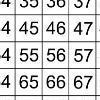### That Number Square!

##### Age 5 to 11Challenge Level

Exploring the structure of a number square: how quickly can you put the number tiles in the right place on the grid?### Light the Lights

##### Age 5 to 7Challenge Level

Investigate which numbers make these lights come on. What is the smallest number you can find that lights up all the lights?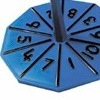### Our Numbers

##### Age 5 to 7Challenge Level

These spinners will give you the tens and unit digits of a number. Can you choose sets of numbers to collect so that you spin six numbers belonging to your sets in as few spins as possible?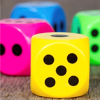### Dicey Operations

##### Age 11 to 14Challenge Level

Who said that adding, subtracting, multiplying and dividing couldn't be fun?### Nice or Nasty

##### Age 7 to 14Challenge Level

There are nasty versions of this dice game but we'll start with the nice ones...### Coded Hundred Square

##### Age 7 to 11Challenge Level

This 100 square jigsaw is written in code. It starts with 1 and ends with 100. Can you build it up?### What Do You Need?

##### Age 7 to 11Challenge Level

Four of these clues are needed to find the chosen number on this grid and four are true but do nothing to help in finding the number. Can you sort out the clues and find the number?##### Age 7 to 14Challenge Level

Watch our videos of multiplication methods that you may not have met before. Can you make sense of them?### Diagonal Sums

##### Age 7 to 14Challenge Level

In this 100 square, look at the green square which contains the numbers 2, 3, 12 and 13. What is the sum of the numbers that are diagonally opposite each other? What do you notice?### How Many Miles to Go?

##### Age 11 to 14Challenge Level

How many more miles must the car travel before the numbers on the milometer and the trip meter contain the same digits in the same order?### What an Odd Fact(or)

##### Age 11 to 14Challenge Level

Can you show that 1^99 + 2^99 + 3^99 + 4^99 + 5^99 is divisible by 5?### Trebling

##### Age 7 to 11Challenge Level

Can you replace the letters with numbers? Is there only one solution in each case?### Sixty-seven Squared

##### Age 16 to 18Challenge Level

Evaluate these powers of 67. What do you notice? Can you convince someone what the answer would be to (a million sixes followed by a 7) squared?### Six Times Five

##### Age 11 to 14Challenge Level

How many six digit numbers are there which DO NOT contain a 5?### Which Is Quicker?

##### Age 7 to 11Challenge Level

Which is quicker, counting up to 30 in ones or counting up to 300 in tens? Why?### Think of Two Numbers

##### Age 11 to 14Challenge Level

Think of two whole numbers under 10, and follow the steps. I can work out both your numbers very quickly. How?### Reach 100

##### Age 7 to 11Challenge Level

Choose four different digits from 1-9 and put one in each box so that the resulting four two-digit numbers add to a total of 100.### All the Digits

##### Age 7 to 11Challenge Level

This multiplication uses each of the digits 0 - 9 once and once only. Using the information given, can you replace the stars in the calculation with figures?### Number Rules - OK

##### Age 14 to 16Challenge Level

Can you produce convincing arguments that a selection of statements about numbers are true?### Two and Two

##### Age 11 to 16Challenge Level

How many solutions can you find to this sum? Each of the different letters stands for a different number.### Which Scripts?

##### Age 7 to 11Challenge Level

There are six numbers written in five different scripts. Can you sort out which is which?### Plus Minus

##### Age 14 to 16Challenge Level

Can you explain the surprising results Jo found when she calculated the difference between square numbers?### Legs Eleven

##### Age 11 to 14Challenge Level

Take any four digit number. Move the first digit to the end and move the rest along. Now add your two numbers. Did you get a multiple of 11?### Big Powers

##### Age 11 to 16Challenge Level

Three people chose this as a favourite problem. It is the sort of problem that needs thinking time - but once the connection is made it gives access to many similar ideas.### 2-digit Square

##### Age 14 to 16Challenge Level

A 2-Digit number is squared. When this 2-digit number is reversed and squared, the difference between the squares is also a square. What is the 2-digit number?### Writing Digits

##### Age 5 to 7Challenge Level

Lee was writing all the counting numbers from 1 to 20. She stopped for a rest after writing seventeen digits. What was the last number she wrote?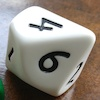### Less Is More

##### Age 5 to 11Challenge Level

Use your knowledge of place value to try to win this game. How will you maximise your score?### The Number Jumbler

##### Age 7 to 14Challenge Level

The Number Jumbler can always work out your chosen symbol. Can you work out how?### Being Curious - Lower Primary Number

##### Age 5 to 7

Number problems to spark your curiosity.### Song Book

##### Age 7 to 11Challenge Level

A school song book contains 700 songs. The numbers of the songs are displayed by combining special small single-digit cards. What is the minimum number of small cards that is needed?### Being Resilient - Primary Number

##### Age 5 to 11Challenge Level

Number problems at primary level that may require resilience.### Being Resourceful - Primary Number

##### Age 5 to 11Challenge Level

Number problems at primary level that require careful consideration.### Being Collaborative - Primary Number

##### Age 5 to 11Challenge Level

Number problems at primary level to work on with others.### Being Curious - Primary Number

##### Age 5 to 11Challenge Level

Number problems for inquiring primary learners.### Reversals

##### Age 11 to 14Challenge Level

Where should you start, if you want to finish back where you started?##### Age 11 to 14Challenge Level

What happens when you add a three digit number to its reverse?##### Age 11 to 14Challenge Level

By selecting digits for an addition grid, what targets can you make?### More Upper Primary Activities on Number Sense and Place Value

##### Age 7 to 11

More upper primary number sense and place value tasks.### More Lower Primary Number Sense and Place Value Activities

##### Age 5 to 7

More activities which will help you get a better of sense of numbers and understand what we mean by place value.### Amount

##### Age 5 to 11

These tasks will help learners develop their understanding of place value, particularly giving them opportunities to express numbers as amounts.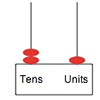### Position

##### Age 5 to 11

One of the key ideas associated with place value is that the position of a digit affects its value. These activities support children in understanding this idea.